# Move the Matches Puzzles Episode 2 Level 1-20 Walkthrough

Walkthrough for all the solutions and answers to the game Move the Matches Puzzles by K-Lab. Get the app on the Google Play Store to play along if you do not already have it.

### Level 1Instructions: Move 3 matches to get 3 triangles

### Level 2Instructions: Remove 1 match to get the correct equation

Solution: 4-1=3

### Level 3Instructions: Move 1 match to get the correct equation

Solution: 6-5=1

### Level 4Instructions: Remove 1 match to get the correct equation

Solution: 6/3=2

### Level 5Instructions: Move 3 matches to get 2 squares

### Level 6Instructions: Move 2 matches to get 3 triangles

### Level 7Instructions: Move 2 matches to get 4 squares, all equal

### Level 8Instructions: Remove 1 match to get the correct equation

Solution: 7×9=63

### Level 9Instructions: Move 1 match to get the correct equation

Solution: 6×3=18

### Level 10Instructions: Move 2 matches to get 4 squares

### Level 11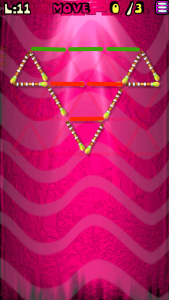Instructions: Move 3 matches to get 3 triangles

### Level 12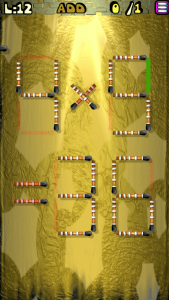Instructions: Add 1 match to get the correct equation

Solution: 4×9=36

### Level 13Instructions: Add 2 matches to get the correct equation

Solution: 7+9=16

### Level 14Instructions: Remove 4 matches to get 3 squares

### Level 15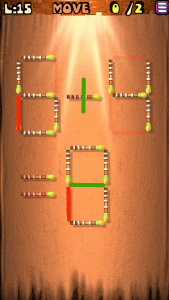Instructions: Move 2 matches to get the correct equation

Solution: 5+4=9

### Level 16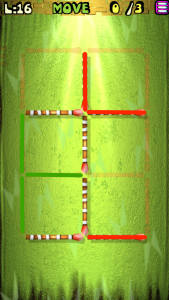Instructions: Move 3 matches to get 2 squares

### Level 17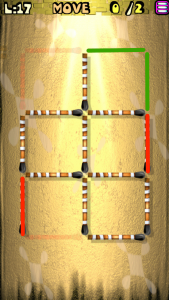Instructions: Move 2 matches to get 3 squares

### Level 18Instructions: Add 2 matches to get the correct equation

Solution: 6+8=14

### Level 19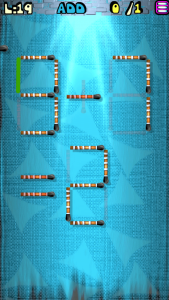Instructions: Add 1 match to get the correct equation

Solution: 9-7=2

### Level 20Instructions: Move 2 matches to get the correct equation

Solution: 2+8=10